Practice the questions of McGraw Hill Math Grade 4 Answer Key PDF Chapter 2 Lesson 3 Adding Whole Numbers to secure good marks & knowledge in the exams.

Question 1.Explanation:
Sum of 491 and 564 we get 1,055.

Question 2.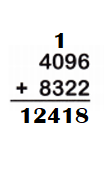Explanation:
Sum of 4096 and 8322 we get 12,418.

Question 3.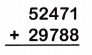Explanation:
Sum of 52,471 and 29,788 we get 82,259.

Question 4.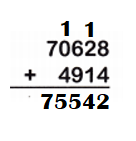Explanation:
Sum of 70,628 and 4,914 we get 75,542.

Question 5.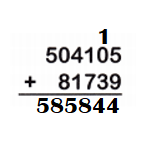Explanation:
Sum of 504,105 and 81,739 we get 585,844.

Question 6.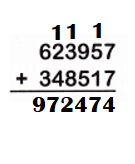Explanation:
Sum of 623,957 and 348,517 we get 972,474.

Question 7.
38,231 + 25,877 = ___________
Sum of 38,231 and 25,877 we get 64,108.

Explanation:
38,231 + 25,877 = 64,108.

Question 8.
620,873 + 76,455 = ____
Sum of 620,873 and 76,455 we get 697,328.

Explanation:
620,873 + 76,455 = 697,328.

Question 9.
4,564 + 98,100 = __________
Sum of 4,564 and 98,100 we get 102,664.

Explanation:
4,564 + 98,100 = 102,664.

Question 10.
408,641 + 449,243 = ____
Sum of 408,641 and 449,243 we get 857,884.

Explanation:
408,641 + 449,243 = 857,884.

Question 11.
Look at problem 7 above. Is regrouping needed in the final step? Why or why not?